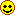Examples
Here are some calculations what I made and from what I build transformers.
1. Transformer: 220V - 2x27V 8A
Input voltage 220 Volts.
Output voltage two times by 27 Volts 8 Ampere, symmetric for amplifier.
EI core intersection square is 50 by 48 millimeters.
Calculated values:
• Input 220 Volts 2.258 Ampere
• 443 coils of 1.05 millimeters wire thickness
Here I use wire of 1 millimeter, because core is big enough and warming at that thickness is low. True reason is that I didn't have wire of 1.05 millimeters.• Output 2 x 27 Volts 8 Ampere
• 2 x 54 coils of 1.98 millimeters round to 2 millimeters.
In assembling I use for output two wires of 2 millimeters at one quill.
Beginning point of two wires are joined and this is output ground, end point is two outputs of 27 Volts.
I build that transformer and it works perfectly.